# CMAT Quantitative Aptitude Practice Questions Set-3

CMAT Quantitative Aptitude Practice questions Set-3: This practice set has 10 questions with their answers covering topics from the areas of Quantitative Aptitude.

Dec 9, 2015 18:24 IST

CMAT Quantitative Aptitude Practice questions Set-3: This practice set has 10 questions with their answers covering topics from the areas of Quantitative Aptitude. This Practice set includes the Questions as per the CMAT Exam pattern and syllabus.

1. 1/2 and 1/4 quantities of alcohol are there in two similar glasses. They are filled with water to make the glass full. What is the ratio of water to alcohol in the new mixture?

a) 3:5
b) 5:3
c) 4:4
d) 3:2

2. What is the diagonal of a rectangle with perimeter and area, 32m and 56 m2 respectively?

a) 144 m
b) 12 m
c) 13
d) 16

3. Find the marks obtained by two students in an examination such that the marks secured by one of them were 10 more than the other and his marks was 60% of the sum of their marks.

a) 33, 43
b) 21, 31
c) 30, 20
d) 42, 52

4. Find out the price at which Rahul buy the suitcase if he bought a suitcase with 15% discount on the labelled price and he sold the suitcase with 20% profit on the labelled price for Rs.2880?

a) Rs.2040
b) Rs.2400
c) Rs.2604
d) Rs.2640

5. If a2 + 1/a2 = 14 then the value of a2 – a + 1/a will be?

a) ± 3
b) ± 5
c) 3, – 5
d) – 3, 5

6. If two dice are thrown simultaneously then the probability that the sum is neither 6 nor 8?

a) 5/18
b) 13/18
c) 7/18
d) 11/18

7. There is a rectangular garden having dimension 20m × 30m. There is a jogging track inside the garden along its perimeter. The width of the track is uniform and it is equal to 2m. Find the total perimeter of the jogging track and the area of the jogging track?

a) 184 m2
b) 202 m2
c) 236 m2
d) 156 m2

8. A can complete a work working 4 hours a day in 5 days and B can complete the same work in 5 days working 4 hours a day. If both work together for 8 hours a day, how many days will it take to complete the job?

a) 10
b)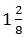c)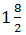d) 1

Directions (9 -10): Study the table below and answer the questions given below:

 S No Company Expenditure 2009-10 (cr) Expenditure 2010-11 (cr) 1 A 1.25 1.62 2 B 1.42 1.87 3 C 1.85 2.12 4 D 1.76 2.04 5 E 2.21 2.45

9. What is the percentage increase in expenditure of all the companies together during 2011 than during 2010?

a) 19.68%
b) 16.89%
c) 18.96%
d) 18.69%

10. Which of the company has the least percentage increase in expenditure in 2011?

a) A
b) C
c) D
d) E

1. (b), 2. (b), 3. (c), 4. (a), 5. (d), 6. (b), 7. (a), 8. (b), 9. (c), 10. (d)

Explanations:

Explanation 1:

Let the glasses be A and B.
A contains 1/2 of alcohol and the remaining 1/2 of water.
B contains 1/4 of alcohol and 3/4 of water.
Proportion of alcohol = 1/2 + 1/4 = 3/4
Proportion of water= 1/2 + 3/4 = 5/4
Ratio of water to alcohol is 5:3

Explanation 2:

Let the diagonal of the rectangle be d.
Area, lb= 56 m2
Perimeter, P = 2(l + b)= 32m
Squaring P2 = 4(l + b)2
So, P2/4 = (l + b)2
P2/4= l2 + b2 + 2lb
Since d2 = l2 + b2 and Area a= lb
P2/4 = d2 + 2a
d = √(p2/4) - 2a
d = 12m

Explanation 3:

Let their marks be (x + 10) and x.
Then, (x + 10) = 60/100 (x + 10 + x) ⇔ 25(x + 10) = 15(2x + 10) ⇔5 x = 100 ⇔ x = 20.
So, their marks are 30 and 20.

Explanation 4:

Let labelled price be Rs. X. Then, 120% of x = 2880
⇒    x = (2880 × 100/120) = 2400.
∴     C.P. = 85% of Rs.2400 = Rs. (85/100 × 2400) = Rs. 2040.

Explanation 5: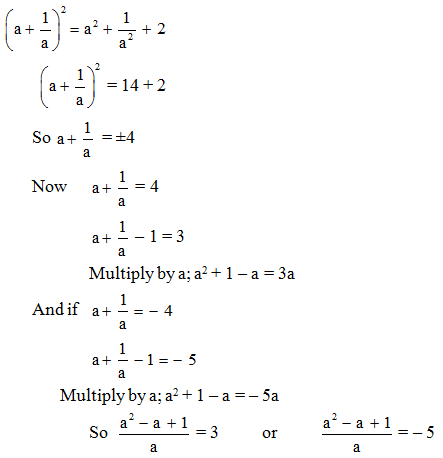Explanation 6:

Suppose A be the event of getting the sum 6 or 8. Then
A= { (1,5) ,(2,4) , (3,3) , (4,2) , (5,1) , (2,6) , (3,5) , (4,4) , ( 5,3) , (6,2) }
Now on throwing the two dice total number of outcomes = 6 × 6 = 36
Number of outcomes of getting the sum 6 or 8 = 10
So, the probability of getting the sum 6 or 8 = 10/36 = 5/18
Now, the required probability = 1 – p (A) = 1 – 5/18 = 13/18

Explanation 7:

ABCD is the rectangular garden and the shaded in it is the jogging track.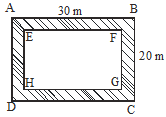Perimeter of the jogging track = Perimeter of ABCD + Perimeter of EFGH
EF = 30 – (2 × 2) = 26 m because the width of the track is 2 m on either side.
Similarly, FG = 20 – (2 × 2) = 16,
Perimeter = 2(30 + 20) + 2(26 + 16) = 100 + 84 = 184 m
Area of the jogging track = (30 × 20) – (26 × 16) = 600 – 416 = 184 m2

Explanation 8:

A works (4*5)= 20 hours
B works (5*4)= 20 hours
Work done by A in 1 hour= 1/20
Work done by B in 1 hour= 1/20
A and B’s one hour’s work= 1/20 + 1/20 = 1/10
So A and B can together work for 10 hours to finish the job
10 hours (in terms of 8 hours per day) =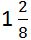days

Explanation 9:

Total expenditure during 2010 is 8.49 cr
Total expenditure during 2011 is 10.1 cr
Difference is 10.1 - 8.49 = 1.61 cr
Percentage increase= 1.61/8.49 × 100 = 18.96%

Explanation 10:

Percentage Increase in expenditure of company A = 29.6%
Percentage Increase in expenditure of company C = 14.5%
Percentage Increase in expenditure of company D = 15.9%
Percentage Increase in expenditure of company E = 10.8%